# AP Physics 2 Practice Test 10

### Test Information10 questions18 minutes

1.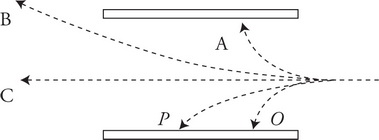A beam of various particles is launched into the space between two oppositely charged parallel plates as shown in the figure. It is known that the particles are all traveling at the same initial velocity as they enter the region, and that particle P is a proton. What else can be inferred?

2. The figure shows an electric potential field created by charges that are not shown.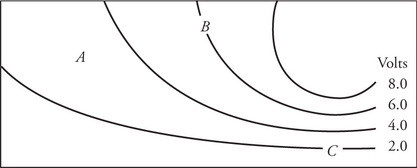A small negative charge is placed at the location labeled A. Which one of the following vectors most closely depicts the direction of the electric force on the charge?

3. The figure shows an electric potential field created by charges that are not shown.At which location is the electric field strongest, and which direction is the electric field?

4.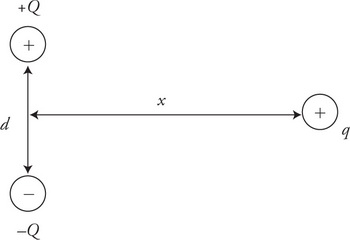Two spheres with charges +Q and –Q of equal magnitude are placed a vertical distance d apart on the y-axis as shown in the figure. A third charge +q is brought from a distance x, where x >> d, horizontally toward the midpoint between +Q and –Q. The net force on +q as it is moved to the left along the x-axis:

5.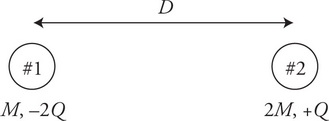Two charges are separated by a distance D as shown in the figure. Charge #1, on the left, has a mass of M and a charge of −2Q. Charge #2, on the right, has a mass of 2M and a charge of +Q. The two charges are released. Where will the charges collide and why?

6.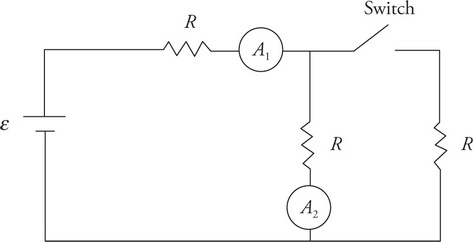The circuit shown has a battery of emf ε; three identical resistors, R; two ammeters, A1 and A2; and a switch that is initially in the open position as shown in the figure. When the switch is closed, what happens to the current reading in the two ammeters?

A1     A2

7.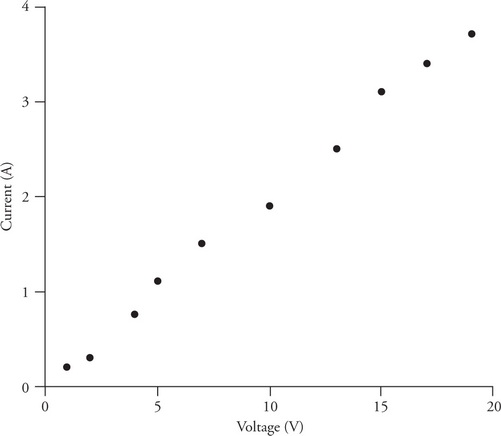A kitchen toaster is connected to a variable power supply. The voltage difference across and current through the toaster are measured for various settings of the power supply. The figure shows the graph of the data. The resistance of the toaster:

8. The figure shows three resistors, X, Y, and Z, which have different shapes but are made of the same material. The resistors as shown are connected to a voltage source.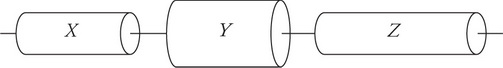Which of the following correctly ranks the current in the resistors?

9. The figure shows three resistors, X, Y, and Z, which have different shapes but are made of the same material. The resistors as shown are connected to a voltage source.Which of the following correctly ranks the potential difference across the resistors?

10.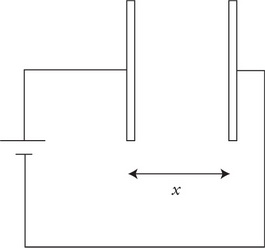A capacitor with movable parallel plates is connected to a battery. The plates of the capacitor are originally a distance x apart as shown in the figure. With the battery still connected, what happens to the energy stored in the capacitor and the electric field between the capacitor plates, if the plates are moved to a new distance x/2?

Energy     Electric Field